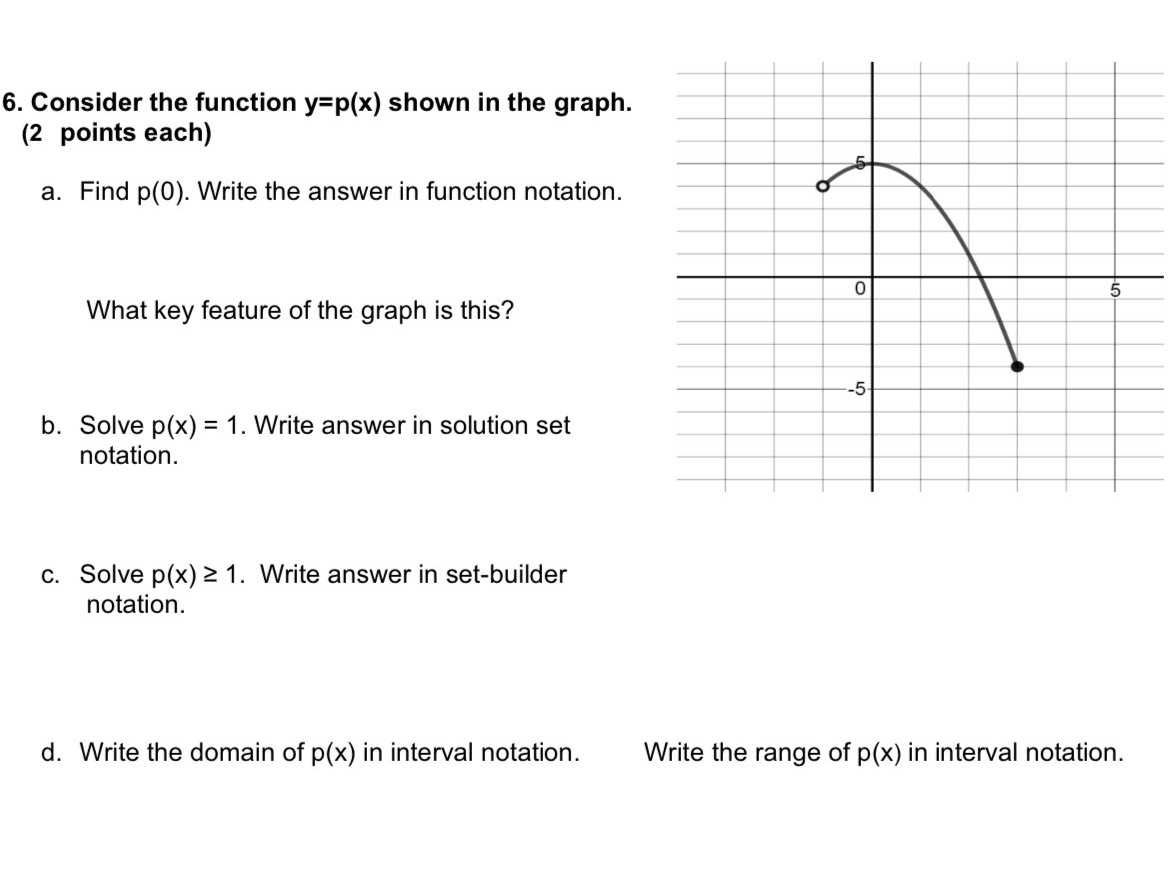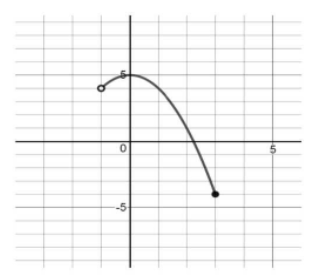### ¿Todavía tienes preguntas de matemáticas?

Pregunte a nuestros tutores expertos
Algebra
Pregunta6. Consider the function $$y = p ( x )$$ shown in the graph. (2 points each)

a. Find $$p ( 0 )$$ . Write the answer in function notation.

What key feature of the graph is this?

b. Solve $$p ( x ) = 1$$ . Write answer in solution set notation.

c. Solve $$p ( x ) \geq 1$$ . Write answer in set-builder notation.

d. Write the domain of $$p ( x )$$ in interval notation. Write the range of $$p ( x )$$ in interval notation.$$5$$
$$2$$
$$(- 1,2]$$
$$(- 1,3]$$
$$[- 4,5]$$